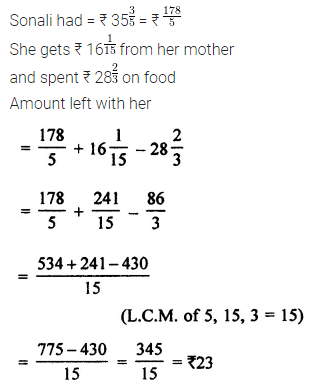# ML Aggarwal Class 7 Solutions for ICSE Maths Chapter 2 Fractions and Decimals Ex 2.2

## ML Aggarwal Class 7 Solutions for ICSE Maths Chapter 2 Fractions and Decimals Ex 2.2

Question 1.
Evaluate the following: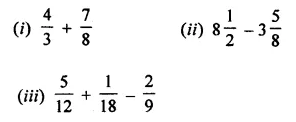Solution: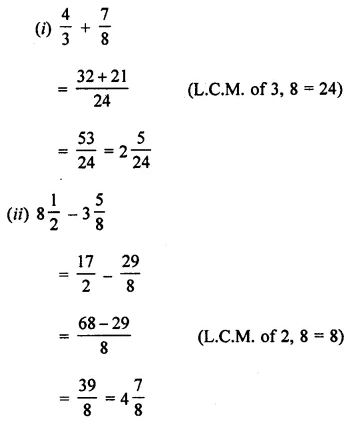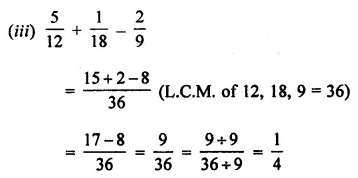Question 2.
Simplify the following: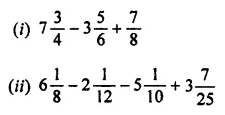Solution: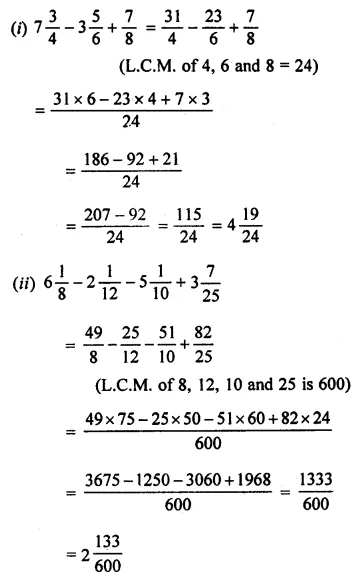Question 3.
Jaishree studies for 5$$\frac { 2 }{ 3 }$$ hours daily. She devotes 2$$\frac { 4 }{ 5 }$$ hours of her time for Science and Mathematics. How much time does she devote to other subjects?
Solution:Question 4.
Ramesh solved $$\frac { 2 }{ 7 }$$ part of an exercise while Reshma solved $$\frac { 4 }{ 5 }$$ of it. Who solved the lesser part? By how much?
Solution: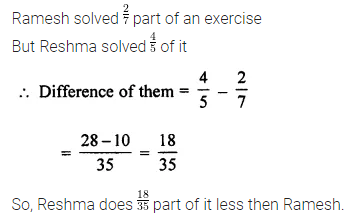Question 5.
Sonali had ₹ 35$$\frac { 3 }{ 5 }$$. She got ₹ 16$$\frac { 1 }{ 15 }$$ from her mother and spent ₹ 28$$\frac { 2 }{ 3 }$$ on food. How much money is left with her?
Solution: